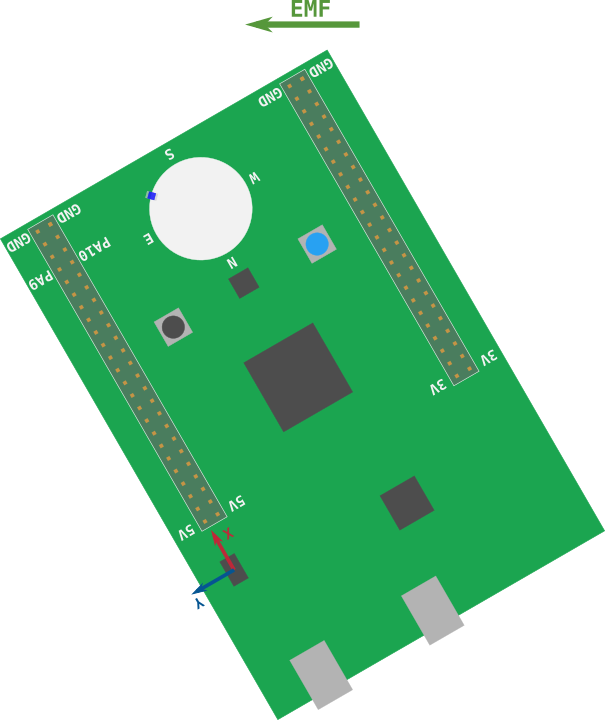# Take 1

What's the simplest way in which we can implement the LED compass? Even if it's not perfect.

For starters, we'd only care about the X and Y components of the magnetic field because when you look at a compass you always hold it in horizontal position thus the compass is in the XY plane.

For example, what LED would you turn on in the following case. EMF stands for Earth's Magnetic Field and green arrow has the direction of the EMF (it points north).The `Southeast` LED, right?

What signs do the X and Y components of the magnetic field have in that scenario? Both are positive.

If we only looked at the signs of the X and Y components we could determine to which quadrant the magnetic field belongs to.In the previous example, the magnetic field was in the first quadrant (x and y were positive) and it made sense to turn on the `SouthEast` LED. Similarly, we could turn a different LED if the magnetic field was in a different quadrant.

Let's try that logic. Here's the starter code:

``````#![deny(unsafe_code)]
#![no_main]
#![no_std]

#[allow(unused_imports)]
use aux15::{entry, iprint, iprintln, prelude::*, switch_hal::OutputSwitch, Direction, I16x3};

#[entry]
fn main() -> ! {
let (leds, mut lsm303dlhc, mut delay, _itm) = aux15::init();
let mut leds = leds.into_array();

loop {
let I16x3 { x, y, .. } = lsm303dlhc.mag().unwrap();

// Look at the signs of the X and Y components to determine in which
// quadrant the magnetic field is
let dir = match (x > 0, y > 0) {
(true, true) => Direction::Southeast,
(false, true) => panic!("TODO"),
There's a `Direction` enum in the `led` module that has 8 variants named after the cardinal points: `North`, `East`, `Southwest`, etc. Each of these variants represent one of the 8 LEDs in the compass. The `Leds` value can be indexed using the `Direction` `enum`; the result of indexing is the LED that points in that `Direction`.